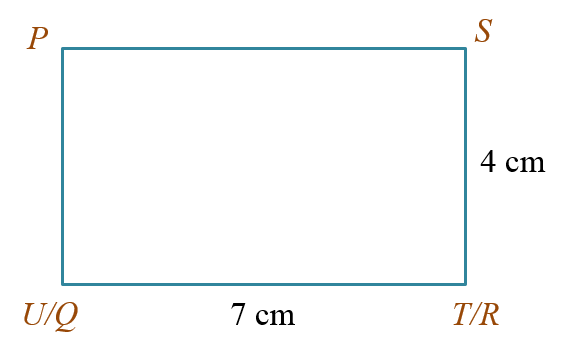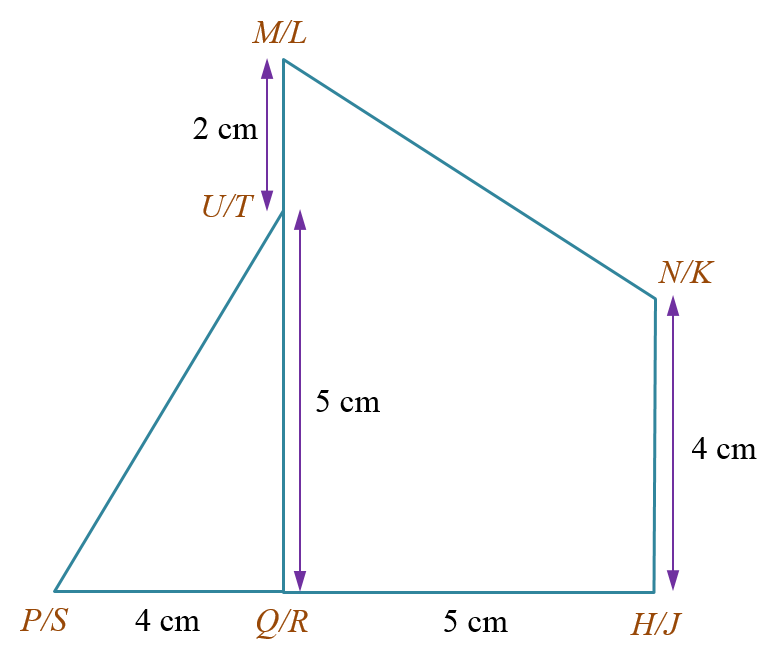# SPM Mathematics 2019, Paper 2 (Question 15)

Question 15:
You are not allowed to use graph paper to answer this question.
(a) Diagram 9.1 shows a solid prism with a rectangular base PQRS on a horizontal plane. The right-angled triangle PQU is the uniform cross section of the prism. The rectangle PUTS is an inclined plane. Edges QU and RT are vertical.Diagram 9.1

Draw to full scale, the plan of the solid. [3 marks]

(
b) Another solid cuboid with rectangular base QHJR and trapezium HNMQ is joined to the prism in Diagram 9.1 at the vertical plane QRTU. Trapezium HNMQ is the uniform cross section of the prism. The composite solid is as shown in Diagram 9.2. The base PQHJRS lies on a horizontal plane. PQH is collinear.Diagram 9.2

Draw to full scale,

(i) the elevation of the composite solid on a vertical plane parallel to PQH as viewed from X. [4 marks]

(ii) the elevation of the composite solid on a vertical plane parallel to HJ as viewed from Y. [5 marks]

Solution:
(a)(b)(i)(b)(ii)